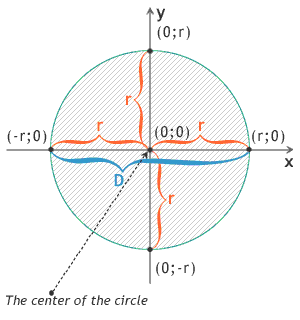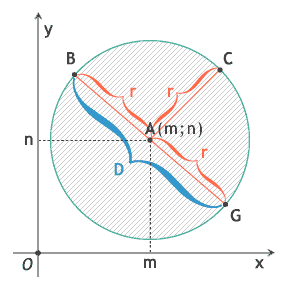# What Is a Circle

What is a circle? Any circle is a geometric figure. You can get it in the geometric plane with the canonical inequality:

x2 + y2 ≤ r2

where r - is radius of the circle, D = 2r - is diameter of the circleor

(x - m)2 + (y - n)2 ≤ r2

where A(m;n) - is the centre

This is an inequality of the circle with the centre in the point A(m;n).Calculators